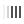# Pressure converting calculator in bars per MPa, kgf and psi

Pressure is a quantity that is equal to the force acting strictly perpendicular to the unit surface area. Calculated by the formula: P = F/S. The international system of calculation assumes the measurement of such a value in pascals (1 Pa is equal to a force of 1 newton per area of ​​1 square meter, N / m2). But since this is a fairly small pressure, measurements are more often indicated in kPa or MPa. In various industries, it is customary to use their own numbering systems; in automotive, pressure can be measured: in bars, atmospheres, kilograms of force per cm² (technical atmosphere), mega pascals or pounds per square inch (psi).

To quickly convert units of measurement, you should focus on this relationship of values to each other:

1 MPa = 10 bar;

100 kPa = 1 bar;

1 bar ≈ 1 atm;

3 atm = 44 psi;

1 PSI ≈ 0.07 kgf/cm²;

1 kgf/cm² = 1 at.

Pressure Ratio Table
Volume MPa bar atm kgf/cm² psi at
1 MPa 1 10 9,8692 10,197 145,04 10.19716
1 bar 0,1 1 0,9869 1,0197 14,504 1.019716
1 atm (physical atmosphere) 0,10133 1,0133 1 1,0333 14,696 1.033227
1 kgf/cm² 0,098066 0,98066 0,96784 1 14,223 1
1 PSI 0,006894 0,06894 0,068045 0,070307 1 0.070308
1 at (technical atmosphere) 0.098066 0.980665 0.96784 1 14.223 1

## Why pressure unit conversion calculator is needed

The online calculator allows you to quickly and accurately convert values ​​from one pressure measurement unit to another. Such a conversion can be useful for car owners when measuring compression in the engine, when checking the pressure in the fuel line, pumping tires to the required value (very often you have to convert PSI to atmosphere or MPa to bar when checking pressure), filling the air conditioner with freon. Since the scale on the gauge can be in one system of calculation, and in the instruction in a completely different one, there is often a need to convert bars to kilograms, megapascals, kilograms of force per square centimeter, technical or physical atmospheres. Or, if you need a result in the English system of calculation, then the pound-force per square inch (lbf • in²), in order to exactly comply with the required instructions.

## How to use online calculator

In order to use sudden converting of one pressure value to another and find out how much a bar will be in MPa, kgf/cm², atm or psi:

1. In the left list, select the unit with which you want to perform the conversion;
2. In the right list, set the unit to which the conversion will be performed;
3. Immediately after entering the number in any of the two fields appears «result». So you can convert from one value to another or vice versa.

For example, in the first field, the number 25 was entered, then, depending on the unit chosen, you calculate how many bars, atmospheres, megapascals, kilograms of force produced per cm² or pounds-force per square inch. When this same value was put in another (right) field, the calculator will say the inverse ratio of the selected physical pressure values.

## FAQ

• ### 1 bar how many atmospheres?

To get an approximate result of how many atmospheres there are in one bar, divide the pressure value by a factor of 1.013. That is, 1 bar is 0.98 atm. Therefore, when converting one unit of measurement of a small pressure (up to 10 bar) to another, it is customary to assume that 1 bar ≈ 1 atm. This ratio will give an error in calculations not exceeding 2%.

• ### How many bars is 1 MPa?

To find out how many bars are in one megapascal, it is sufficient to multiply the pressure value expressed in MPa by 10. That is, 1 MPa = 10 bar.

• ### 1 MPa is how many kgf cm2?

To convert one Megapascal into a pressure value expressed in kilogram-force per square centimeter, it is sufficient to multiply the MPa value by 10.197. Thus 1 MPa = 10.197 kgf/m².

• ### Kgf how many atmospheres?

When converting kgf/cm2 to atm you need to divide the pressure value expressed in kgf cm2 by 1.033. Using this ratio, you can convert any pressure value expressed in kilograms of force to atmospheres.

Loading comments